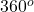## Describe the process for taking a set of data and creating a circle graph from it.

Question

Describe the process for taking a set of data and creating a circle graph from it.

in progress 0
1 month 2021-08-14T06:58:00+00:00 1 Answers 2 views 0

The set of data given is summed so as to determine the total value. Then each data in the set is expressed as a ration of the total value, which is multiplied by. This is to determine the degree of angles that represent each data in the data set. These angles in degrees can now be used to divide the sum of angles in a circle into wedges.